# 图解： EXPLAIN 实战-1

0收藏

### 介绍

``explain select * from teacher``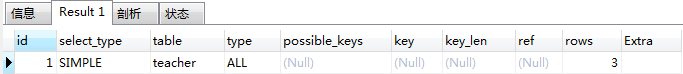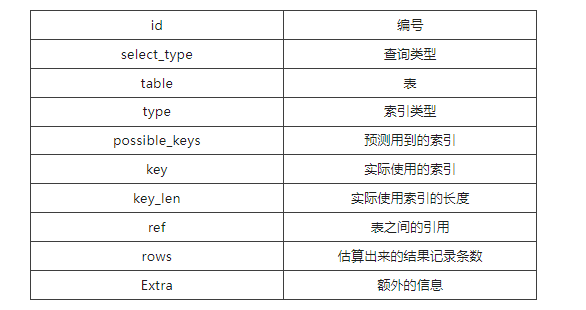course表

``````CREATE TABLE `course` (
`cid` int(3) NOT NULL,
`cname` varchar(20) NOT NULL,
`tid` int(3) NOT NULL,
PRIMARY KEY (`cid`) USING BTREE
) ENGINE=InnoDB DEFAULT CHARSET=utf8mb4;``````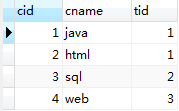teacher表

``````CREATE TABLE `teacher` (
`tid` int(3) NOT NULL,
`tname` varchar(20) NOT NULL,
`tcid` int(3) NOT NULL,
PRIMARY KEY (`tid`)
) ENGINE=InnoDB DEFAULT CHARSET=utf8mb4;``````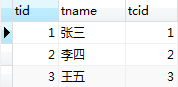teacher_card表

``````CREATE TABLE `teacher_card` (
`tcid` int(3) NOT NULL,
`tcdesc` varchar(20) NOT NULL,
PRIMARY KEY (`tcid`)
) ENGINE=InnoDB DEFAULT CHARSET=utf8mb4;``````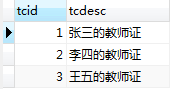### id

``````SELECT
t.*
FROM
course c,
teacher t,
teacher_card tc
WHERE
c.tid = t.tid
AND t.tcid = tc.tcid
AND ( c.cid = 2 OR t.tcid = 3 )``````

explain上述SQL后，如下所示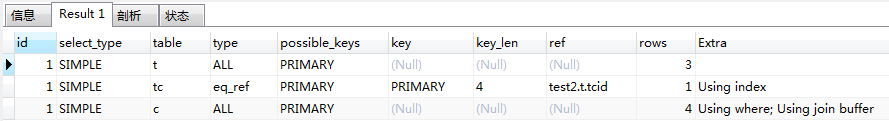id值相同，从上往下顺序执行

``````SELECT
t.*
FROM
course c,
teacher t,
teacher_card tc
WHERE
c.tid = t.tid
AND t.tcid = tc.tcid
AND c.cid = 2``````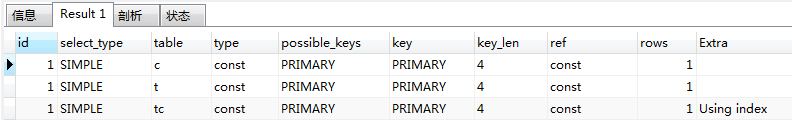``````SELECT
tc.tcdesc
FROM
teacher_card tc
WHERE
tc.tcid = (
SELECT
t.tcid
FROM
teacher t
WHERE
t.tid = ( SELECT c.tid FROM course c WHERE c.cname = "sql" )
);``````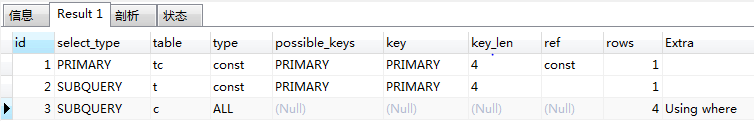id值不同，id值越大，越优先执行。将上述SQL改为如下形式

``````SELECT
tc.tcdesc
FROM
teacher t,
teacher_card tc
WHERE
tc.tcid = t.tcid
AND t.tid = ( SELECT c.tid FROM course c WHERE c.cname = "sql" )``````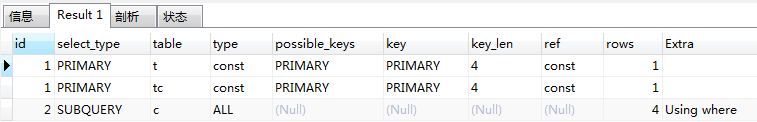id值有相同，又有不同，id值越大越优先，id值相同，从上往下顺序执行

### select_type

SIMPLE：查询不包含子查询和UNION

``SELECT * FROM teacher``PRIMARY：查询有任何复杂的子部分，则外层部分标记为PRIMARYSUBQUERY：包含在SELECT列表中的子查询中的SELECT（换句话说，不在FROM子句中）标记为SUBQUERY

``````SELECT
tc.tcdesc
FROM
teacher_card tc
WHERE
tc.tcid = (
SELECT
t.tcid
FROM
teacher t
WHERE
t.tid = ( SELECT c.tid FROM course c WHERE c.cname = "sql" )
);``````DERIVED：DERIVED值用来表示包含在FROM子句的子查询中的SELECT，MySQL会递归执行并将结果放到一个临时表中。服务器内部称其“派生表”，因为该临时表是从子查询中派生来的

``````SELECT
cr.cname
FROM
( SELECT * FROM course WHERE tid IN ( 1, 2 ) ) cr``````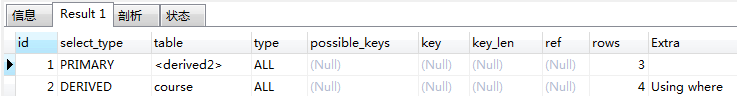id为1的table表名为<derived2>，表示是一张派生表，派生表从id为2的执行过程中来

UNION：在UNION中的第二个和随后 的SELECT被标记为UNION。第一个SELECT被标记就好像它以部分外查询来执行。这就是下面第一个例子中在UNION中的第一个SELECT显示为PRIMARY的原因。如果UNION被FROM子句中的子查询包含，那么它的第一个SELECT会被标记为DERIVED，即下面的第二个例子

UNION RESULT：用来从UNION的匿名临时表检索结果的SELECT被标记为UNION RESULT

``SELECT * FROM course WHERE tid = 1 UNION SELECT * FROM course WHERE tid = 2``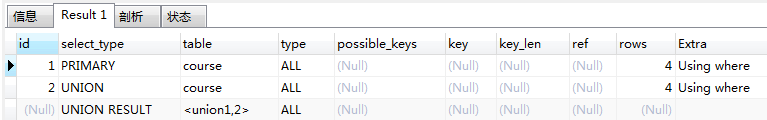``````SELECT
cr.cname
FROM
( SELECT * FROM course WHERE tid = 1 UNION SELECT * FROM course WHERE tid = 2 ) cr``````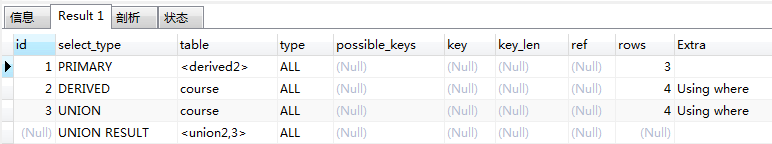### type

system：只有一条数据的系统表，或派生表只有一条数据的子查询

``````SELECT
a.tname
FROM
( SELECT * FROM teacher t WHERE t.tid = 1 ) a``````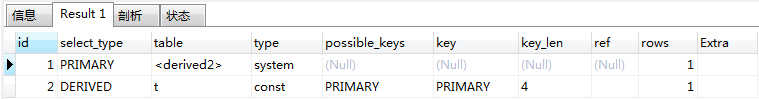const：仅仅能查到一条数据的SQL，用于Primary key或unique索引。如果只是能查到一条数据，但是条件列上没有Primary key或unique索引，则不是const

``SELECT * FROM teacher t WHERE t.tid = 1``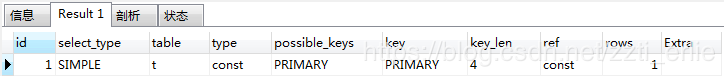``SELECT * FROM teacher t WHERE t.tid = 1``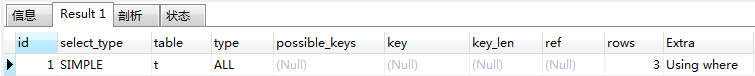eq_ref：唯一性索引，对于每个键的查询，返回匹配唯一行数据（有且只有一个，不能多，不能0），常见于唯一索引和主键索引，不是必须的有索引，我下面句的例子中teacher表的tcid字段就没有加任何索引，当然是我数据太简单的问题，才能在不建索引的情况下出现eq_ref

``````SELECT
t.tcid
FROM
teacher t,
teacher_card tc
WHERE
t.tcid = tc.tcid``````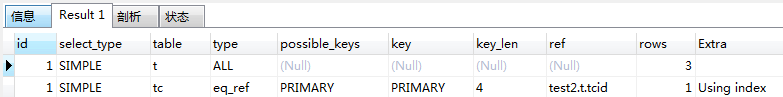teacher表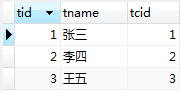teacher_card表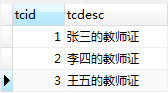teacher表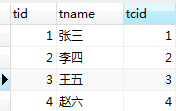``````SELECT
t.tcid
FROM
teacher t,
teacher_card tc
WHERE
t.tcid = tc.tcid``````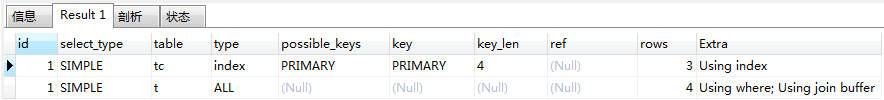ref：非唯一性索引，对于每个索引键的查询，返回匹配所有行（0，多）

teacher表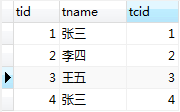``````SELECT
*
FROM
teacher
WHERE
tname = "张三"``````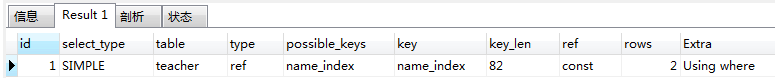range：检索指定范围的行，where后面是一个范围查询（between，>，<，>=，in有时候会失效，从而转为无索引ALL）

``SELECT * FROM teacher WHERE tid < 3``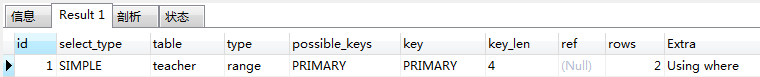index：这个跟全表扫描一样，只是MySQL扫描表时按索引次序进行而不是行

``SELECT tname FROM teacher``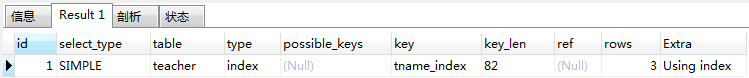``SELECT tname, tcid FROM teacher``

``SELECT tname, tcid FROM teacher``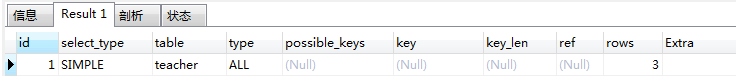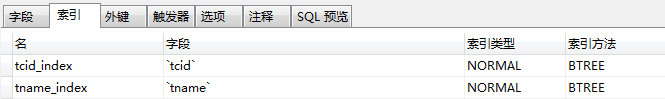``SELECT tname, tcid FROM teacher``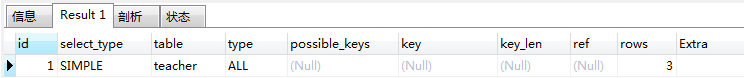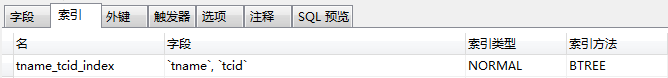``SELECT tname, tcid FROM teacher``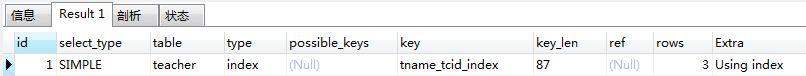key使用了联合索引

all：全表扫描

``SELECT * FROM teacher WHERE tcid = 1``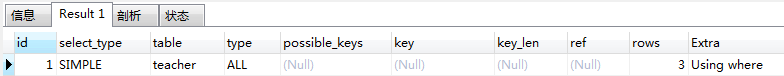### key_len

​索引的长度，用于判断联合索引是否被完全使用，单开一文来讲

### ref

``````SELECT
c.cid,
t.tname
FROM
course c,
teacher t
WHERE
c.tid = t.tid
AND t.tname = "张三"``````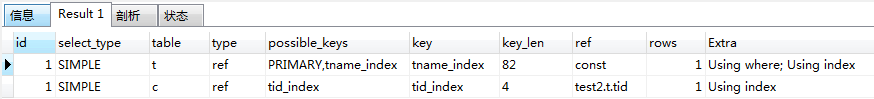### Extra

Using index：所需要的数据，只需要在索引即可全部获得，而不需要再到表中取数据

Using where：如果我们不是读取表的所有数据，或者不是仅仅通过索引就可以获取所有需要的数据，则会出现Using where信息。

Using indexUsing where前面已经有例子，当出现索引覆盖时，会显示Using index，性能得到了提升，出现Using temporary和Using filesort说明性能损耗比较大

Using temporary：当MySQL某些操作中必须使用临时表时，在Extra信息中就会出现Using temporary。主要常见于GROUP BY和ORDER BY等操作中

Using filesort：这意味着MySQL会对结果使用一个外部索引排序，而不是按索引次序从表里读取行

``SELECT * FROM teacher WHERE tname = "张三" ORDER BY tname``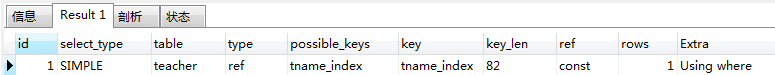``SELECT * FROM teacher WHERE tname = "张三" ORDER BY tcid``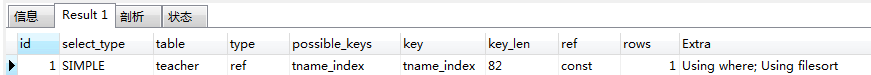帖子
视频
声望
粉丝
最近发布
热门推荐
社区精华内容